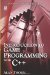# 7.5 Logical Operators

## 7.5 Logical Operators

Logical operators are useful for combining two or more conditional statements into one. So far, this chapter has considered only individual conditional statements, such as (A > B), (A < D), (A == E), and so on. The following logical operators can be used to combine such statements: AND (&&), OR (||), and NOT (!). So, to determine whether variable X is within the range of 0 to 10, two conditional statements can be combined using logical operators as follows: (X >= 0) && (X <= 10). This whole expression will evaluate to true or false, depending on whether X falls within this range. The following list examines the logical operators more closely.

• AND &&

Determines whether any conditional statements are true. This entire statement will only evaluate to true if all conditional statements are true; otherwise it will evaluate to false.

• OR ||

Determines whether any one of a given set of conditional statements is true. If so, the statement will evaluate to true; otherwise it is false if all conditional statements are false.

• NOT!

Determines whether the inverse of a condition is true.

 Note Logical operators in the context of AND were seen briefly in the section on chained inequalities in Chapter 2.

### 7.5.1 If Else Statements and Logical Operators

Since logical operators like AND and OR can be combined with conditional statements like (A > B), they can both affect If statements and If Else statements. Using logical operators with conditional statements means that several conditions can be specified in a single If statement. For example, if((a>b)&&(a<d)). The following code uses If Else statements with conditional and logical operators.

`      #include <iostream>      int main()      {         int Number = 0;         std::cout<<"Enter a number\n";         std::cin >> Key;         if(Number < 10)         {            std::cout<<"Number less than 10\n";         }         if((Number >= 10) && (Number <= 30))         {            std::cout<<"Number from 10 to 30";         }         if(Number != 7)         {            std::cout<<"Number is not 7\n";         }         if((Number == 5) || (Number < 3))         {            std::cout<<"Number is equal to 5 or less than 3\n";         }         return 0;      } `Introduction to Game Programming with C++ (Wordware Game Developers Library)
ISBN: 1598220322
EAN: 2147483647
Year: 2007
Pages: 225
Authors: Alan Thorn

Similar book on Amazon

flylib.com © 2008-2017.
If you may any questions please contact us: flylib@qtcs.net